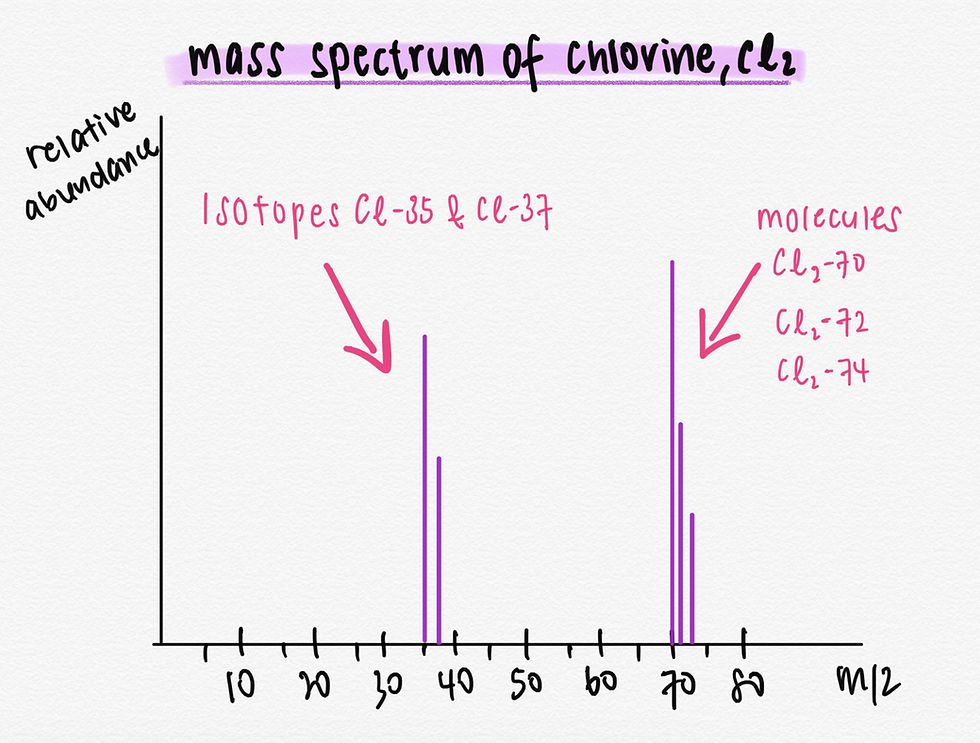top of page
Search

# Mass Spectrometry of Chlorine - O Level Chemistry

You may have come across the question about mass spectrometry of chlorine in pure chemistry exams. Many of us will not understand how to approach when attempting such question. In today’s EduBlog post, we will be explaining how it works and how to answer a typical exam questions that is commonly tested in schools’ exams.In the above graph, we can see the mass spectrum of chlorine molecules giving us five different mass peaks (35, 37, 70, 72, 74).

Before I explain how we get these five different mass peaks, let us first understand some important concepts that will help you to comprehend later on how we get those mass peaks:

Mass spectrometry gives accurate information on the relative masses of isotopes and their relative abundance (proportions).

How mass spectrometer works?Chlorine molecules pass through a mass spectrometer which is an instrument that involves vaporising atoms or molecules in high vacuum and subjecting the vaporised particles to electron bombardment to generate a beam of positive ions, a process called ionisation.

So the mass spectrometer is an instrument for separating ionised/charged (+) particles of different mass and determine the amounts of each particle in a mixture.

What is m/z?

Another concept that we need to know is:

m/z = relative molecular mass or isotopic mass / electric charge for ions formed in a mass spectrometer

In short, it’s mass/charge ratio.

For example, [35-Cl2]+ has a relative mass of 70 and a positive ion charge of +1, the m/z ratio is 70/1 = 70.

Understanding why there are different mass peaks

Now we will explain simply why there are five different mass peaks for chlorine molecules.

When chlorine molecules passes through the ionisation chamber, an electron is loss from the molecules, to give positively charged molecular ion ( Cl2+ ). Cl2+ ions are not stable so they will fall apart to give Cl atoms and Cl+ ions.

This is called Fragmentation.

The Cl atoms formed will not get ionised in the chamber so they get lost in the machine (neither accelerate nor deflected). Then the Cl+ will pass through the machine to give 35 and 37 because chlorine consists of two principal stable isotopes, chlorine-37 (~25% is 37Cl) and chlorine-35 (~75% is 35Cl).

The problem is that the unfragmented Cl2+ ions still exist. So we need to think about the possible combination of chlorine-35 and chlorine-37 atoms in Cl2+ ions.

That will give you a total masses of Cl2+ ion of:

35 + 35 = 70

35 + 37 = 72

37 + 37 = 74

This gives an expected ratio of the molecular ions 70 : 72 : 74 of 9 : 6 : 1, and this is what you observe for peaks 3 to 5.

Now, let us see an example from past year school prelim question on mass spectrometry of Chlorine.As you can see from the figure 8.2, we have to find the values of x and y.

Are you able to answer both of them?

Here are the answer to them:That's about it for Mass Spectrometry of Chlorine! If you have further questions on this, feel free to leave your questions in the comments section down below. Otherwise, feel free to SMS/Whatsapp us at 9374 9138 should you require any form of help in Chemistry or any other subjects!